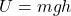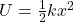Question

A mass is hanging on the end of a spring and oscillating up and down. There are three forces acting on the mass, the force of the spring, the force of gravity and the force of air resistance. Which of these forces can be associated with potential energy function? a. The spring force only. b. Gravity only. c. Air resistance only. d. The spring force and gravity. e. The spring force and air resistance. f. Gravity and air resistance. g. All three forces.

1.thuthao

d. The spring force and gravity.

Explanation:

The forces that can be associated with a potential energy function are only the conservative forces. These are the forces whose work done on an object by the force does not depend on the path taken, but only on the initial and final position of the object.

The only two conservative forces in this problem are:

– Gravity

– The spring force

While the air resistance is a non-conservative force.

The potential energy associated with the gravitational force is:where

m is the mass of the object

g is the acceleration due to gravity

h is the position of the object with respect to a reference level (e.g. the ground)

The potential energy associated to the spring force iswhere:

k is the spring constant

x is the elongation of the spring with respect to the equilibrium position